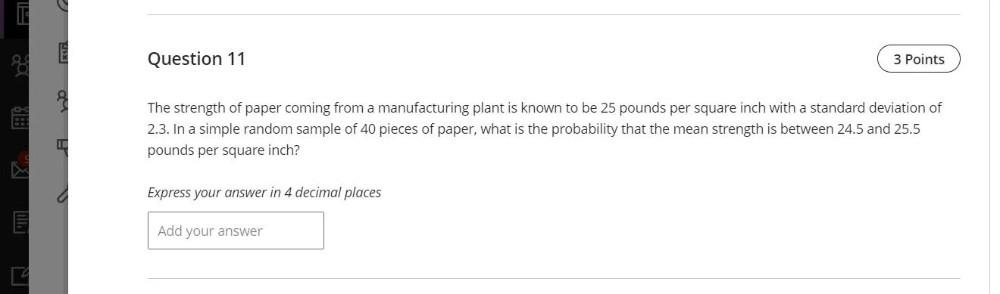Question:

# The strength of paper coming from a manufacturing plant is known to be 25 pounds per square inch with a standard deviation of 2.3. In a simple random sample of 40 pieces of paper, what is the probabilThe strength of paper coming from a manufacturing plant is known to be 25 pounds per square inch with a standard deviation of 2.3. In a simple random sample of 40 pieces of paper, what is the probability that the mean strength is between 24.5 and 25.5 pounds per square inch?Express your answer in 4 decimal places